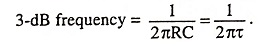## Linear Waveshaping Interview Questions and Answers:

1. What is waveforming?

Ans. Waveforming is a process of generating new waveshape from another waveform using some network.

2. What is meant by linear waveshaping? Give some examples of linear waveshaping circuits.

Ans. Linear waveshaping involves passage of signal through linear systems such as R-C, R-L, R-L-C circuits and the operations involved are linear operations such as integration, differentia­tion, summation, filtering etc.

R-C, R-L and R-L-C circuits are the examples of linear waveshaping circuits.

3. What is difference between linear and nonlinear waveshaping circuits?

Ans. Linear waveshaping involves passage of signal through linear systems such as R-C, R-L, R-L-C circuits and the operations involved are linear operations such as integration, differentia­tion, summation, filtering etc. On the other hand, nonlinear waveshaping involves passage of signal through a nonlinear system. Nonlinear waveshaping permits transformation of analog signal to digital signal and vice versa. The clipping operation and rejection of negative peaks by rectification are nonlinear operations.

4. What is meant by high-pass circuit?

Ans. The circuit that blocks and attenuates low frequencies but allow high-frequency signals to pass through it, is called the high-pass circuit.

5. Why a capacitor in high-pass R-C circuit is named as blocking capacitor?

Ans. Because of its blocking property for dc or low frequency input signals the capacitor acts like an open circuit and blocks the signal and so the capacitor is called the blocking capacitor.

6. What is meant by lower 3-dB frequency of a high-pass circuit?

Ans. Lower 3-dB frequency of a high-pass circuit is the frequency at which capacitive reactance is equal to resistance of the circuit or the frequency at which the output voltage is 0.707 times the maximum value of output voltage.

7. What is relation between 3 dB frequency and time constant in high-pass R-C circuit?

Ans.8. What is meant by a fractional tilt?

Ans. Fractional tilt is defined as the ratio of pulse width (PW) to the time constant τ(RC).

9. What is main reason of applying a step voltage input in a high-pass R-C circuit?

Ans. The main reason of applying a step voltage input in a high-pass R-C circuit is that for times less than the pulse width tthe output voltage is the same as that for a step input because pulse signal is same as step input for t < tp. However, at the end of pulse, as the input becomes zero, the output also drops exponentially to zero. The reason is that capacitor voltage falls exponentially to zero, as the input becomes zero.

10. What is meant by a differentiating circuit?

Ans. A circuit that gives an output voltage proportional to the derivative of its input, is known as a differentiating circuit.

11. What are the applications of high-pass R-C circuits?

Ans. R-C circuit with RC ≫ T is employed in R-C coupling of amplifiers where distortion and differentiation of waveform is to be avoided. R-C circuit with RC << T is employed to generate pips for triggering electronic circuits such as multivibrators, flip-flops etc.

12. What is meant by low-pass circuit?

Ans. Low-pass circuit is that which passes low-frequency signals through it but blocks the high-frequency signals.

13. What is integrator?

Ans. An integrator is that circuit which gives an output voltage directly proportional to the integral of its input.

14. What are the applications of a low-pass R-C circuit?

Ans. R-C circuits with time constant larger than time period of the input signal are used as by-pass capacitors. Low-pass filter circuits are also used in generation of triangular and ramp waveforms. It also finds application in discrimination between pulses of different lengths.

15. What is attenuator?

Ans. Attenuators are the resistive networks, which are used to reduce or attenuate the amplitude of the input signal.

16. Why do resistive attenuators need compensation?

Ans. In order to reduce the rise time due to stray capacitance, attenuators need compensation.

17. What are ringing circuits?

Ans. Ringing circuits are those which generate a sequence of pulses spaced regularly in time.

18. What is the application of ringing circuits?

Ans. Ringing circuits are used in timing operations in automatic control circuits.

19. What is a ringing circuit? Sketch a ringing circuit arrangement with non-zero initial conditions.

Ans. Ringing circuits are those which generate a sequence of pulses spaced regularly in time. A ringing circuit is shown in the figure 3 in which there is an initial voltage V0 across the capacitor as well as an initial inductor current I.

Scroll to Top